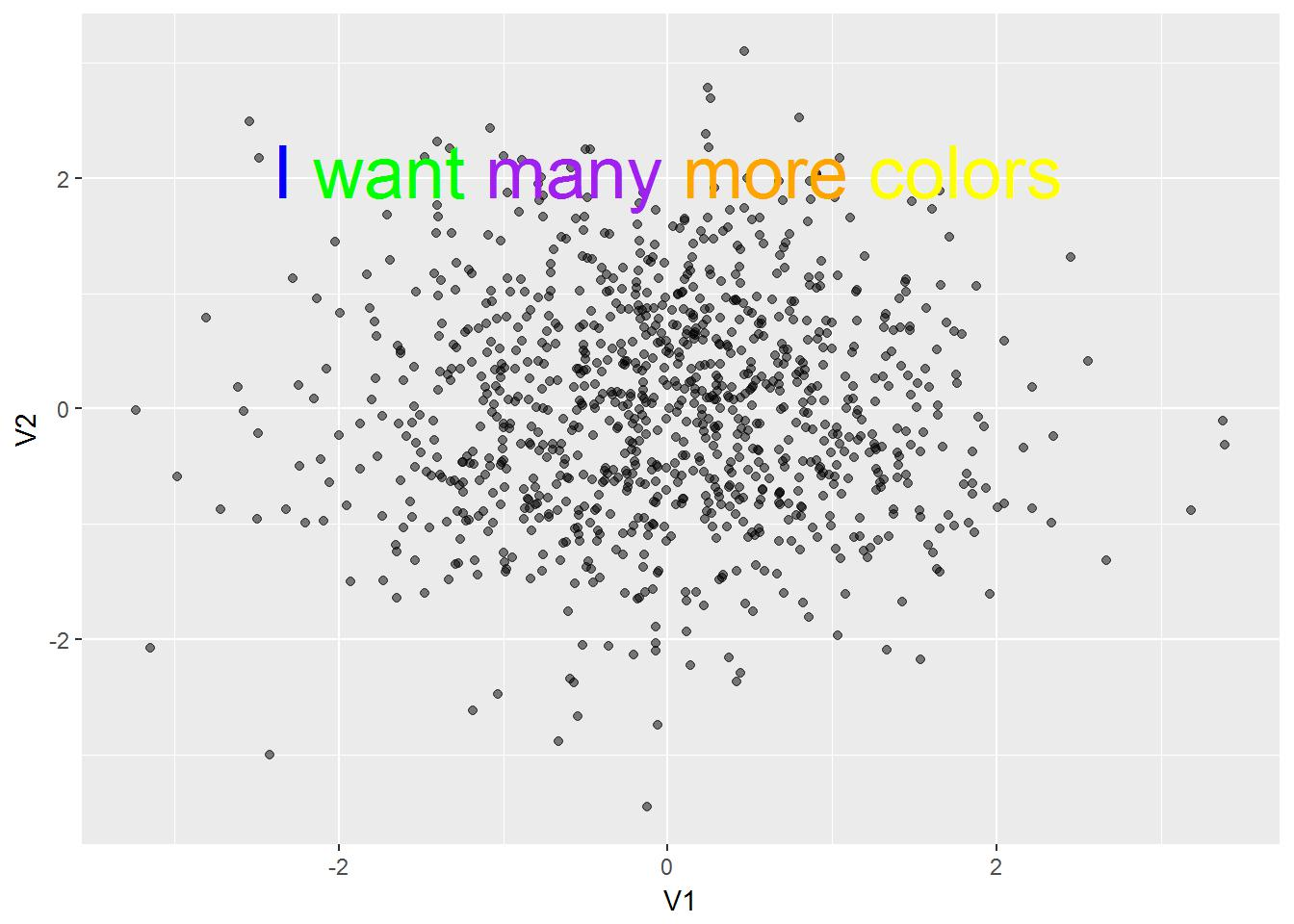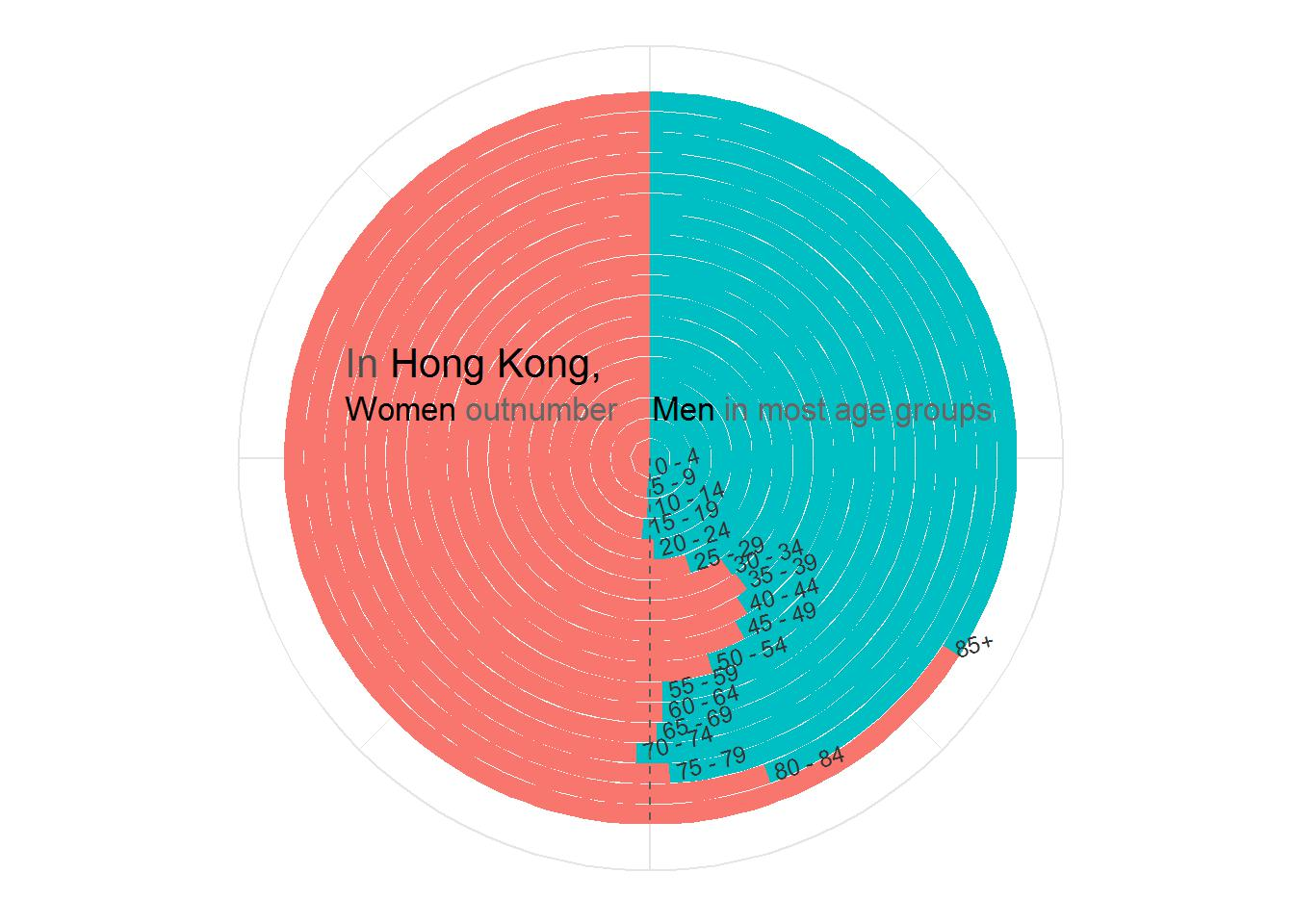If you want to go straight to the function, you can find it at the bottom of the page.

While working on a data visualization project, I discovered that ggplot2 does not allow for easy multicolor plot annoations. So after some searching I discovered this post from Andrew Whitby’s blog. His method is to overlay multiple annotate() functions with plotmath components to hide the parts of strings to allow the strings to overlap. This method requires the text to be formatted as shown below:

``````annotate('text', x = 3, y = .03, parse = T,label = '"I " * phantom("want many more colors")', color = 'grey') +
annotate('text', x = 3, y = .03, parse = T,label = 'phantom("I ") * "want" * phantom("many more colors")', color = 'blue') +
annotate('text', x = 3, y = .03, parse = T,label = 'phantom("I want ") * "many" * phantom("more colors")', color = 'green') +
annotate('text', x = 3, y = .03, parse = T,label = 'phantom("I want many ") * "more" * phantom("colors")', color = 'purple') +
annotate('text', x = 3, y = .03, parse = T,label = 'phantom("I want many more ") * "colors"', color = 'orange') ``````

This code hides the word that isn’t being passed through phantom() and will color it. Then, it stacks the labels onto each other and prints them on the plot. The huge downside of this method is that it is very labor instensive and requires very specific formatting. So I decided to write a function that writes all of the annotate() functions for me.

The function, annotate_color(), takes its arguments in the same order as annotate function and to call the same set of functions you would just type:

``````annotate_color(x = 3, y = .03,
labels = 'I want many more colors',
colors = c('blue', 'green', 'purple', 'orange', 'yellow'))``````

Both of these blocks of code would evaluate to something like this:Hopefully, you won’t actually use it to create a graph that looks like that as it is quite ugly.
This is an example of how it could be better used.### Input methods

``````# Make or import your data
data <- as.tibble(replicate(10, rnorm(1000)))

# Assigning a color for each word
data %>%
ggplot(aes(x = V1, y = V2)) +
geom_point() +
annotate_color(x = 0, y = 2.7, size = 6,
labels = 'Assign different colors for each word',
colors = c('blue', 'green', 'purple', 'orange', 'black', 'yellow'))

# Assigning a color for only one word in a string
data %>%
ggplot(aes(x = V1, y = V2)) +
geom_point() +
annotate_color(x = 0, y = 2.7, default_color = 'black',
labels = 'Assign different colors for one word',
colors = c('', '', 'red')) # You must assign strings before the target word you want to color...
# ... as words are colored in the order you type them

#  Assigning colors for first and last words
data %>%
ggplot(aes(x = V1, y = V2)) +
geom_point() +
annotate_color(x = 0, y = 2.7, default_color = 'purple', #
labels = 'Assign different colors for the first and last words',
colors = c('red', '', '', '', '', '', '', '', 'blue'))

# Coloring only the first word and all of the following words the same color.
data %>%
ggplot(aes(x = V1, y = V2)) +
geom_point() +
annotate_color(x = 0, y = 2.7, default_color = 'Grey30',
labels = 'Assign different colors for one word',
colors = c('red'))

### Notes
# You can pass every argument that annotate() will accept into annotate_color().
# The default geom type for annotate_color() is text, you can change it to any other geom that has a...
# ... 'label' and a 'color'argument.
# 'black' is the default value for 'default_color'``````

### The function

``````## This function will allow you to assign color to each word in a plot annotation
# Dependencies: purrr, ggplot2

annotate_color <- function(geom = 'text', x = NULL, y = NULL, xmin = NULL, xmax = NULL,
ymin = NULL, ymax = NULL, xend = NULL, yend = NULL, ...,
labels = NULL, colors = NULL, default_color = 'black'){

# Checks for essential arguments
if (is.null(colors) || is.null(x) || is.null(y) || is.null(labels)){
stop('Missing one of the arguments: labels, colors, x, or y')}

labels <- strsplit(labels, " ")[]
n <- length(labels)

if (length(colors) < length(labels)){   # Assigns any empty values in 'colors' to the 'default_color'
colors <- map_chr(seq_len(length(labels)), function(i){
if (is.na(colors[i]) | colors[i] == ''){
colors[i] <- default_color
} else {colors[i] <- colors [i]}}
)
}

if (length(colors) > length(labels)){   # Shortens the length of 'colors' to match the length of 'labels'
colors = colors[1:length(labels)]
warning('The length of the colors arg is longer than the number of words in the labels arg. Extra colors will be ignored.')
}

# Formats the labels argument into usable arguments for each annotation function
labels <- map_chr(seq_len(n), function(i) {
start0 <- labels[seq_along(labels) < i]    # Assigns the first part of the string
mid0 <- labels[i]                          # Assigns a single word
end0 <- labels[seq_along(labels) > i]      # Assigns the last part of the string
start <- paste0('phantom("', paste(start0, collapse = " "), ' ")') # Wraps phantom() around the first part
end <- paste0('phantom("', paste(end0, collapse = " "), ' ")') # Wraps phantom() around the last part
if(length(start0) > 0 && length(end0) > 0) {  # Conditional statements for the formatting depending...
paste(start, paste0('"', paste(mid0, collapse = " "), '"'), end, sep = ' * ') # ... on the position of 'mid0'
} else if (length(end0) > 0) {
paste(paste0('"', paste(mid0, collapse = " "), '"'), end, sep = ' * ')
} else if (length(start0) > 0) {
paste(start, paste0('"', paste(mid0, collapse = " "), '"'), sep = ' * ')
} else {
stop("couldn't finish ...")
} # Anonymous function above created with the assistance of
})  # https://stackoverflow.com/users/3521006/docendo-discimus

# Plugs all arguments into the annotate() function and stores them into a list
annofuncs <- list()
annofuncs <- map2(labels, colors, function(annolabel, annocolor){
annofuncs[seq_along(annolabel)] <- list(annotate(geom, x, y, xmin, xmax, ymin, ymax, xend, yend, ...,
parse = T, label = annolabel, color = annocolor))
})
return(annofuncs) # Returns the list which can be added to a ggplot like any other layer
}``````